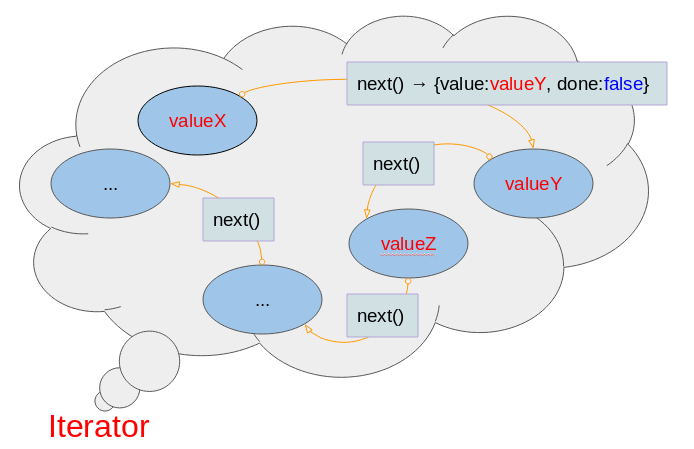# Undertanding JavaScript Iterables and Iterators

## 1. Iteration

ECMAScript 6 provides you with a new way to interact with data structure, which is Iteration. Now, we will clarify it.
There are two concepts that you need to distinguish:
• Iterator
• Iterable
The concepts of Iterator, Iterables apply to the Array, Set, and Map classes.

## 2. Concept of Iterator

Iterator: An object called Iterator if it contains a pointer to next element in Iteration.
Technically, an object in ECMAScript is called an Iterator if it has a method with the next() name, and this method returns an object with the form {value:SomeValue,done:booleanValue}. done has true value if the Iteration has been completed, otherwise it has false value.iterator-object-example.js
``````// An Iterator Object:
let myIterator = {
someProp: "Some Prop",
//
next : function()  {
return  {
value: "Tom",
done: false
}
}
}
// ----------- TEST -------------------
let entry = myIterator.next();
console.log(entry); // { value: 'Tom', done: false }
console.log(entry.value); // Tom``````

## 3. Concept of Iterable

Iterable - In terms of language, an object is called Iterable if it contains a collection of data, and it provides a way to publicize its data. For example, an array can be called Iterable because it contains a collection of data, and you can access its elements.
Technically, in the ECMAScript, an object that is called Iterable must have a method with the key of Symbol.iterator and this method returns an Iterator object
Symbol.iterator is a value of the Symbol data type, like 100 is a value of Integer type. You can see also Symbol in the following post:
Below is a simple example, an object with properties:
• myProp1
• myProp2
• 100
• myProp3
• Symbol.iterator
object-with-properties.js
``````// An Object:
let myObject = {
// A property
myProp1 : "Some Value 1",
// A property
'myProp2' : "Some Value 2",
// A property
100 : "One hundred",
// A property (Method)
myProp3 :  function()  {
console.log("I'm a method");
},
// A property (Method)
[Symbol.iterator] : function() {
console.log("I'm a [Symbol.iterator] method");
}
}
// ----------- TEST --------------
console.log( myObject["myProp1"] ); // Some Value 1
console.log( myObject["myProp2"] ); // Some Value 2
console.log( myObject ); // One hundred
myObject["myProp3"](); // I'm a method
myObject[Symbol.iterator]();// I'm a [Symbol.iterator] method``````
Output:
``````Some Value 1
Some Value 2
One hundred
I'm a method
I'm a [Symbol.iterator] method``````
Iterable?
To sum up, an object is called Iterable if it has a method with a Symbol.iterable key, and this method returns an Iterator object.
iterable-object-example.js
``````// An Iterator Object
let myIterator = {
next : function()  {
return  {value: Math.random(), done: false};
}
}
// A Iterable object:
let myIterable = {
myProp : "Some value",
// A Method returns an Iterator object.
[Symbol.iterator] :  function() {
return myIterator;
}
}
// ------ TEST -----------
// An iterator object.
let it = myIterable[Symbol.iterator]();

let entry = it.next();
console.log(entry);

entry = it.next();
console.log(entry);``````
Output:
``````{ value: 0.6026736348993575, done: false }
{ value: 0.1790056262472559, done: false }``````
A class is called Iterable if it has a method with the [Symbol.iterator] name. The objects created from this class will be Iterable objects.
class-iterable-example.js
``````// An Iterable class.
class MyClass {
constructor() {
}
someMethod() {
console.log("Some method");
}
[Symbol.iterator]() {
// An Iterator object.
var myIterator = {
next: function() {
return {value: Math.random(),done: false};
}
};
return myIterator;
}
}
// ----------- TEST -----------------
let myObject = new MyClass();
myObject.someMethod();
// An Iterator Object.
let it = myObject[Symbol.iterator]();
let entry;
let i = 0;
while ( (entry = it.next()).done == false )  {
console.log( entry);
i++;
if(i > 5)  {
break;
}
}``````
Note: In the ECMAScript, the Array, Set, Map classes are Iterable.